# Force Notes Force A force is a push

• Slides: 20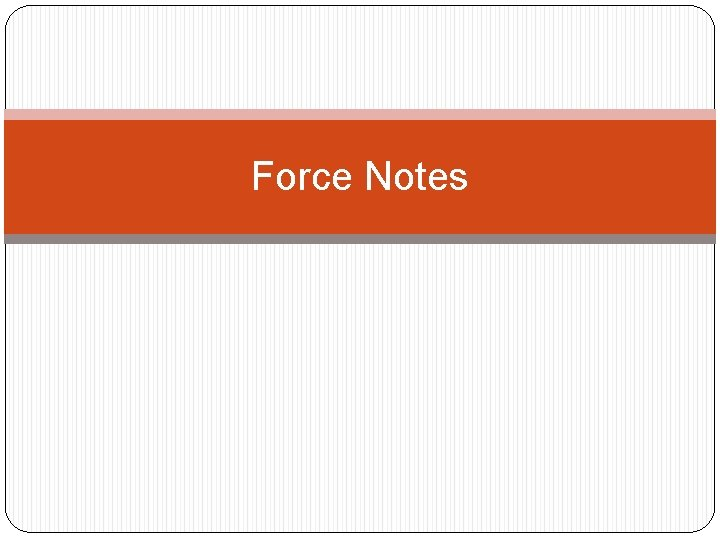Force Notes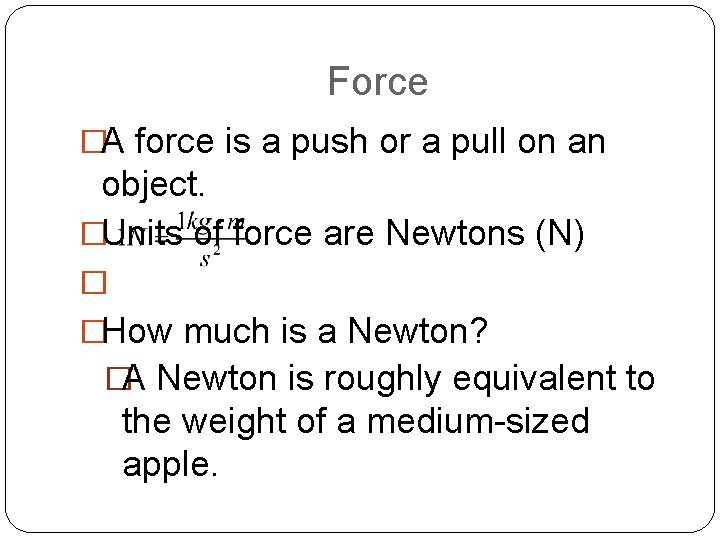Force �A force is a push or a pull on an object. �Units of force are Newtons (N) � �How much is a Newton? �A Newton is roughly equivalent to the weight of a medium-sized apple.Newton’s 1 st Law of Motion �An object at rest will remain at rest, and an object in motion will remain in motion, at constant velocity and in a straight line, unless acted upon by a net force. �(Also known as the Law of Inertia. )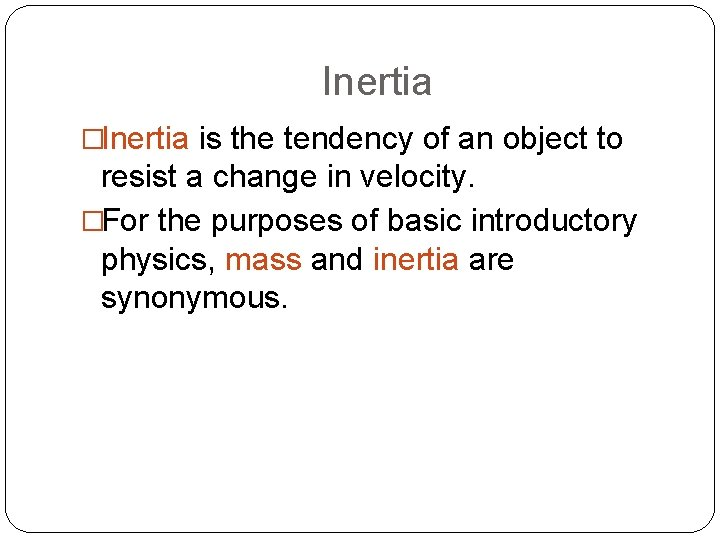Inertia �Inertia is the tendency of an object to resist a change in velocity. �For the purposes of basic introductory physics, mass and inertia are synonymous.Sample Problem – Inertia �Which object has the greatest inertia? A. A falling leaf B. A softball in flight C. A seated high school student D. A helium-filled party balloonWhich object has the greatest inertia? A. A 5 -kg mass moving at 10 m/s B. A 10 -kg mass moving at 1 m/s C. A 15 -kg mass moving at 10 m/s D. A 20 -kg mass moving at 1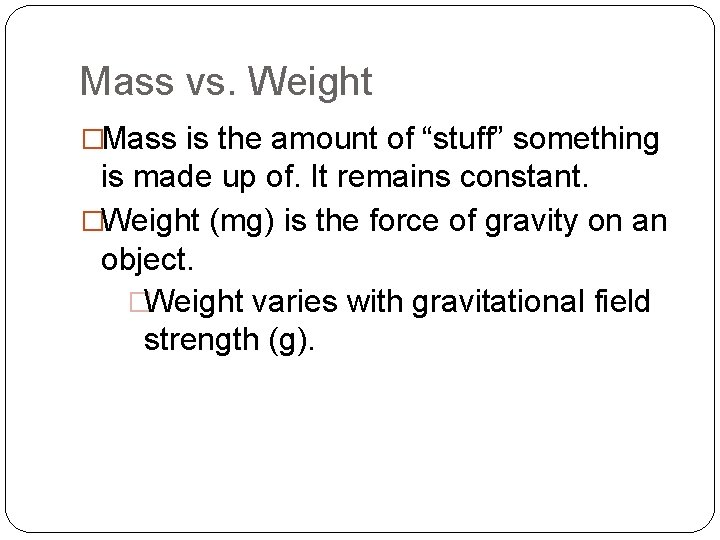Mass vs. Weight �Mass is the amount of “stuff” something is made up of. It remains constant. �Weight (mg) is the force of gravity on an object. �Weight varies with gravitational field strength (g).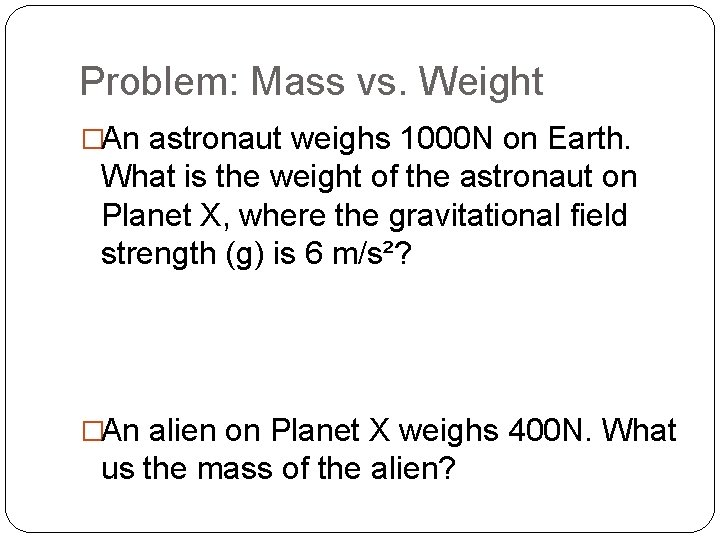Problem: Mass vs. Weight �An astronaut weighs 1000 N on Earth. What is the weight of the astronaut on Planet X, where the gravitational field strength (g) is 6 m/s²? �An alien on Planet X weighs 400 N. What us the mass of the alien?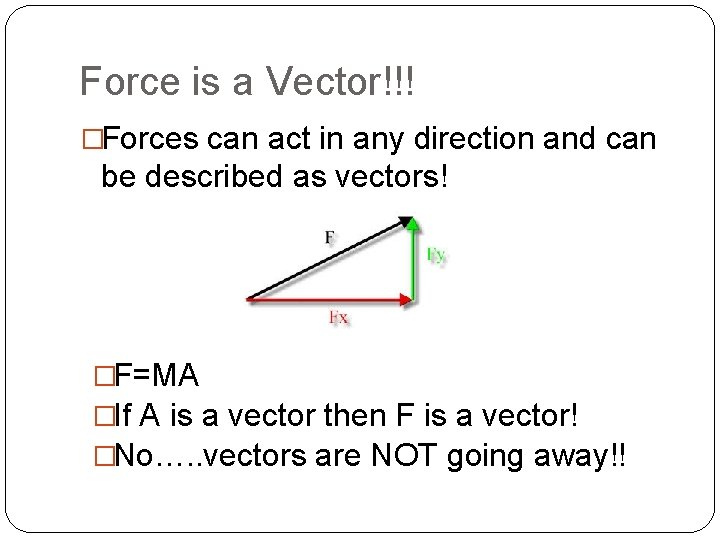Force is a Vector!!! �Forces can act in any direction and can be described as vectors! �F=MA �If A is a vector then F is a vector! �No…. . vectors are NOT going away!!What is a Net Force? �A net force is the vector sum of all the forces acting on an object. �If all forces are balanced, there is no net force. The object is in equilibrium.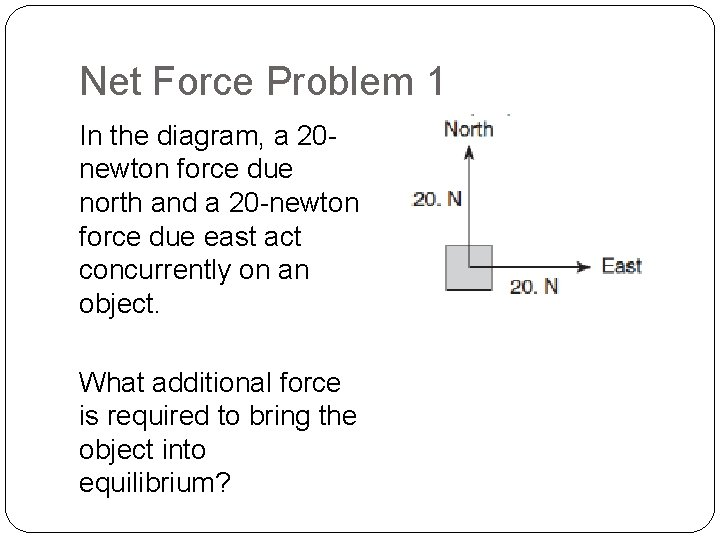Net Force Problem 1 In the diagram, a 20 newton force due north and a 20 -newton force due east act concurrently on an object. What additional force is required to bring the object into equilibrium?Newton’s 2 nd Law of Motion The acceleration of an object is in the direction of and directly proportional to the net force applied, and inversely proportional to the object mass.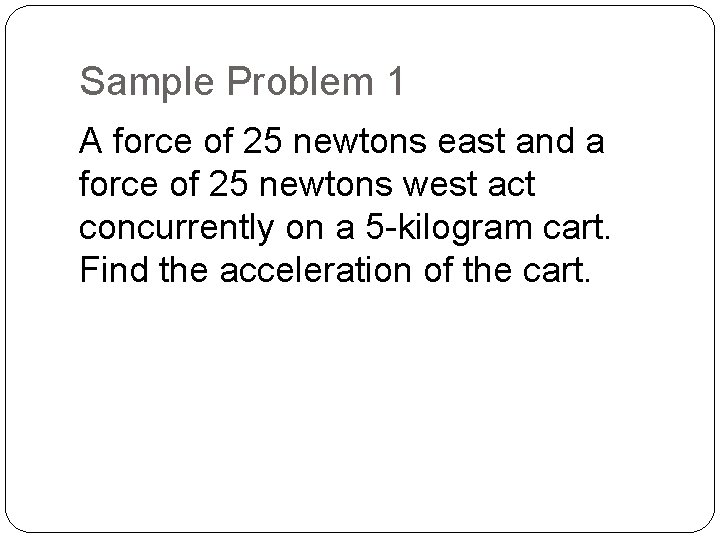Sample Problem 1 A force of 25 newtons east and a force of 25 newtons west act concurrently on a 5 -kilogram cart. Find the acceleration of the cart.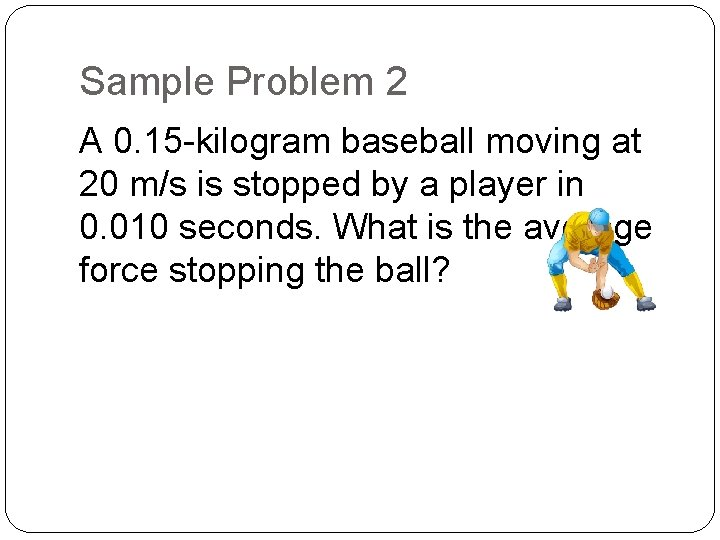Sample Problem 2 A 0. 15 -kilogram baseball moving at 20 m/s is stopped by a player in 0. 010 seconds. What is the average force stopping the ball?Sample Problem 3 Two forces, F 1 and F 2, are applied to a block on a frictionless, horizontal surface as shown below. If the magnitude of the block’s acceleration is 2. 0 meters per second², what us the mass of the block?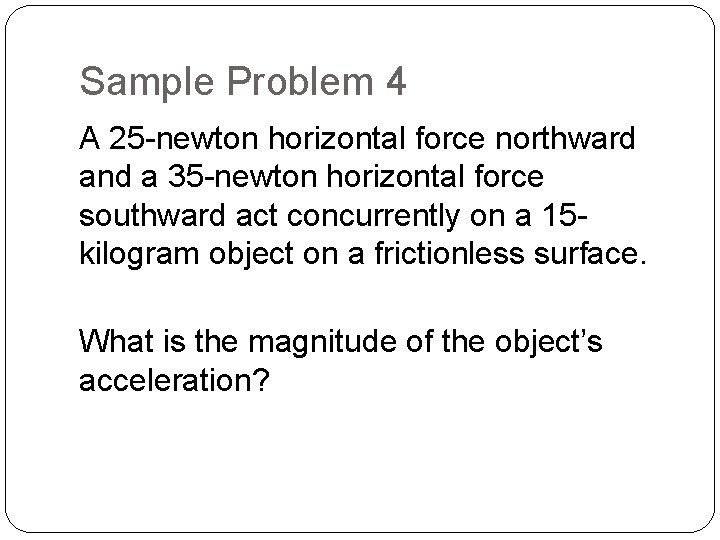Sample Problem 4 A 25 -newton horizontal force northward and a 35 -newton horizontal force southward act concurrently on a 15 kilogram object on a frictionless surface. What is the magnitude of the object’s acceleration?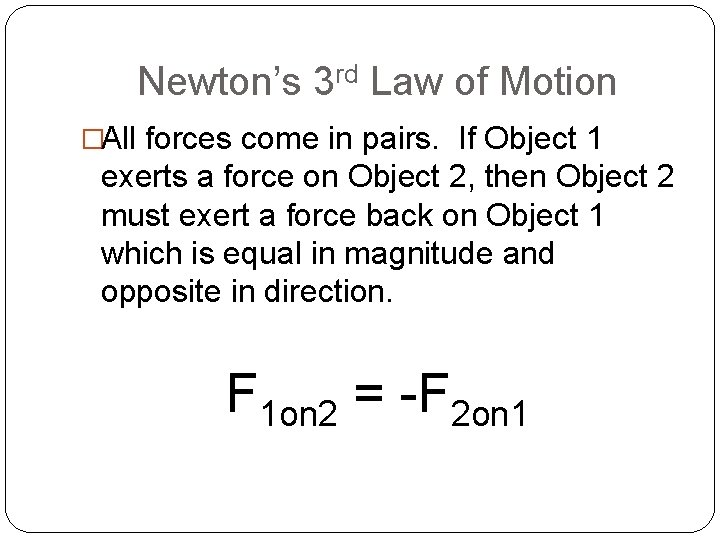Newton’s 3 rd Law of Motion �All forces come in pairs. If Object 1 exerts a force on Object 2, then Object 2 must exert a force back on Object 1 which is equal in magnitude and opposite in direction. F 1 on 2 = -F 2 on 1Sample Problem 1 �Earth’s mass is approximately 81 times the mass of the Moon. If the Earth exerts a gravitational force of magnitude F on the moon, the magnitude of the gravitational force of the Moon on the Earth is a) F b) F/81 c) 9 F d) 81 F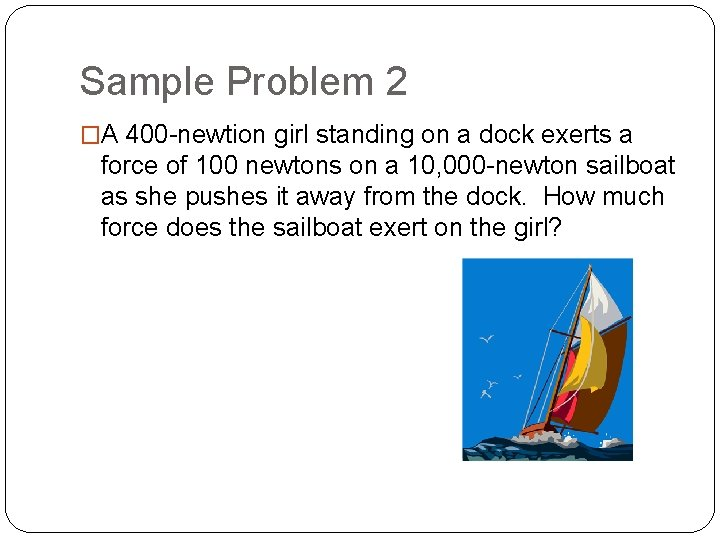Sample Problem 2 �A 400 -newtion girl standing on a dock exerts a force of 100 newtons on a 10, 000 -newton sailboat as she pushes it away from the dock. How much force does the sailboat exert on the girl?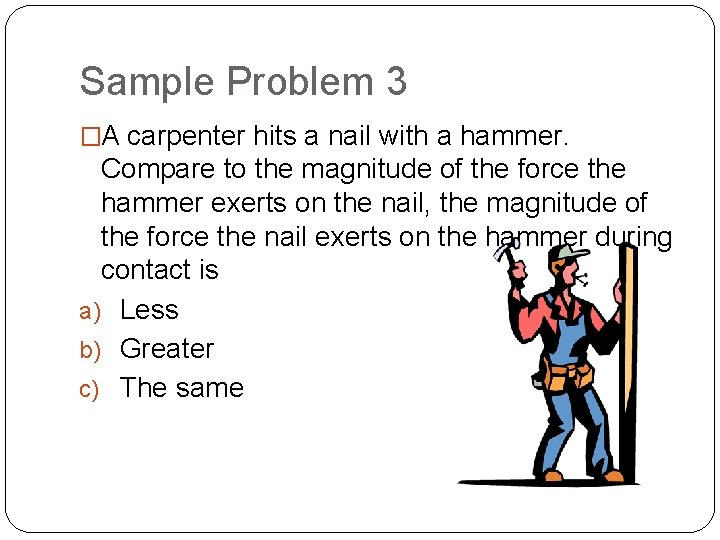Sample Problem 3 �A carpenter hits a nail with a hammer. Compare to the magnitude of the force the hammer exerts on the nail, the magnitude of the force the nail exerts on the hammer during contact is a) Less b) Greater c) The same